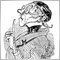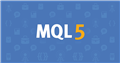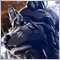# The purpose of return(0) within a if statement within curly bracket359

Hi,

I am trying to figure out  what is the purpose of return(0)?

In my if statement within the curly bracket, i tested with and without retrun(0) in it and the results is the same.

So, does it mean return(0) doesnt really serve any purpose?Moderator
7768

With return you are leaving the function, so it depends how the function is defined!

Jut start reading here: https://www.mql5.com/en/docs/basis/function.Documentation on MQL5: Language Basics / Functions
• www.mql5.com
Every task can be divided into subtasks, each of which can either be directly represented in the form of a code, or divided into smaller sub-tasks. This method is called of the function definition. The function header includes a description of the return value type, name (identifier) and formal parameters.  The number of parameters passed to...359

```if (iTime(Symbol(),PERIOD_H1,0)!=P600520)
{
if (H1Ema5>H1Ema20 && DPH1E520 <=1 )
{
CounterDown600520=0;
CounterUp600520 = CounterUp600520 + 1;
if (H1Ema20 >H1Ema100 && SlopeH1E5>=0 && CounterUp600520==1)
{
Print (Symbol()," : (H11+) H1 E5X20 = ( CU : ",CounterUp600520," : iCH10 : ",iCH10," :  H1Ema5>H1Ema20 : ",NormalizeDouble(H1Ema5,5)," > ",NormalizeDouble(H1Ema20,5));
Print (Symbol()," : (H11+) H1 E5X20 = ( SH1E5 : ",NormalizeDouble(SlopeH1E5,1)," :  SH1E20 : ",NormalizeDouble(SlopeH1E20,1)," : SH1E1008 ： ",NormalizeDouble(SlopeH1E1008,1)," : SH4E1008 ： ",NormalizeDouble(SlopeH4E1008,1)," : ",A," : ",B," : ",C," : ",D," : ",E,")");
P600520=iTime(Symbol(),PERIOD_H1,0);
return(0);
}
}
if (H1Ema5<H1Ema20 && DNH1E520<=1)
{
CounterUp600520=0;
CounterDown600520= CounterDown600520 +1;
if (H1Ema20 <H1Ema100 && SlopeH1E5 <=0 && CounterDown600520==1 )
{
Print (Symbol()," : (H11-) H1 E5X20 = ( CD : ",CounterDown600520," : iCH10 : ",iCH10," :  H1Ema5<H1Ema20 : ",NormalizeDouble(H1Ema5,5)," < ",NormalizeDouble(H1Ema20,5));
Print (Symbol()," : (H11-) H1 E5X20 = ( SH1E5 : ",NormalizeDouble(SlopeH1E5,1)," :  SH1E20 : ",NormalizeDouble(SlopeH1E20,1)," : SH1E1008 ： ",NormalizeDouble(SlopeH1E1008,1)," : SH4E1008 ： ",NormalizeDouble(SlopeH4E1008,1)," : ",A," : ",B," : ",C," : ",D," : ",E,")");
P600520=iTime(Symbol(),PERIOD_H1,0);
return(0);
}
}
}```359

Hi,

Base on the code i post at the top, is it necessary to have the 2 return(0) inside the if statements? Does it serve any purpose?11944

chua le:

really depends upon the function that contains the if statement. You are sending a message to the current function.359

Hi,

I many occasions, i deleted return(0) in the if statement and there is no errors cocuured and yet the output result is the same with that with the return(0) in the if statement.

So it seems to me the return(0) is redundant in these cases.Moderator
16086

chua le:

Hi,

I many occasions, i deleted return(0) in the if statement and there is no errors cocuured and yet the output result is the same with that with the return(0) in the if statement.

So it seems to me the return(0) is redundant in these cases.

The return is put there by the coder for a reason. Because you believe that it is redundant, that doesn't make it so.

In your sample code, we don't know what code follows your snippet, but it seems that if either of the if conditions are satisfied, it is not necessary to execute any more code that may be in the function.

IT is not a good idea to remove returns if you don't know why they are there.359

Thank you Keith,

I read in the forum and it is explained as such :

The point of return/return(0) is to terminate the EA for the current tick. And then, when the next tick arrives, everything inside int start(){} starts all over again until it hits another return.

Is this the right explanation?359

Hi,

The code is  inside the main program to determine the crosiing of Ema5 and Ema 20, if there is crossing, it will be printed in the journal during the backtest. I repost here the codes.

```if (iTime(Symbol(),PERIOD_H1,0)!=P600520)
{
if (H1Ema5>H1Ema20 && DPH1E520 <=1 )
{
CounterDown600520=0;
CounterUp600520 = CounterUp600520 + 1;
if (H1Ema20 >H1Ema100 && SlopeH1E5>=0 && CounterUp600520==1)
{
Print (Symbol()," : (H11+) H1 E5X20 = ( CU : ",CounterUp600520," : iCH10 : ",iCH10," :  H1Ema5>H1Ema20 : ",NormalizeDouble(H1Ema5,5)," > ",NormalizeDouble(H1Ema20,5));
Print (Symbol()," : (H11+) H1 E5X20 = ( SH1E5 : ",NormalizeDouble(SlopeH1E5,1)," :  SH1E20 : ",NormalizeDouble(SlopeH1E20,1)," : SH1E1008 ： ",NormalizeDouble(SlopeH1E1008,1)," : SH4E1008 ： ",NormalizeDouble(SlopeH4E1008,1)," : ",A," : ",B," : ",C," : ",D," : ",E,")");
P600520=iTime(Symbol(),PERIOD_H1,0);
return(0);
}
}
if (H1Ema5<H1Ema20 && DNH1E520<=1)
{
CounterUp600520=0;
CounterDown600520= CounterDown600520 +1;
if (H1Ema20 <H1Ema100 && SlopeH1E5 <=0 && CounterDown600520==1 )
{
Print (Symbol()," : (H11-) H1 E5X20 = ( CD : ",CounterDown600520," : iCH10 : ",iCH10," :  H1Ema5<H1Ema20 : ",NormalizeDouble(H1Ema5,5)," < ",NormalizeDouble(H1Ema20,5));
Print (Symbol()," : (H11-) H1 E5X20 = ( SH1E5 : ",NormalizeDouble(SlopeH1E5,1)," :  SH1E20 : ",NormalizeDouble(SlopeH1E20,1)," : SH1E1008 ： ",NormalizeDouble(SlopeH1E1008,1)," : SH4E1008 ： ",NormalizeDouble(SlopeH4E1008,1)," : ",A," : ",B," : ",C," : ",D," : ",E,")");
P600520=iTime(Symbol(),PERIOD_H1,0);
return(0);
}
}
}```Moderator
16086

chua le:

Thank you Keith,

I read in the forum and it is explained as such :

The point of return/return(0) is to terminate the EA for the current tick. And then, when the next tick arrives, everything inside int start(){} starts all over again until it hits another return.

Is this the right explanation?

Not exactly.

Your own functions may also return a value.

When a function hits a return, it will return to where it was called from.